# CAT 2019 Question Paper | Quants Slot 1

###### CAT Previous Year Paper | CAT Quants Questions | Question 20

Here is a tough question from Arithmetic progressions. As we have already seen with CAT previous year paper, progressions questions are either extremely easy or super tough. This falls into the latter bucket. Try cracking it. The key lies in doing a small operation that will unlock the solution. With good practice you can crack these kind of progressions questions.

Question 20 : If a1, a2, ......... are in A.P , $$frac{1}{\sqrt{a_1} + \sqrt{a_2}}$ + $\frac{1}{\sqrt{a_2} + \sqrt{a_3}}$ + ......... + $\frac{1}{\sqrt{a_n} + \sqrt{a_{n+1}}}$ then , is equal to 1. $\frac{n}{\sqrt{a_1} + \sqrt{a_{n+1}}}$ 2. $\frac{n - 1}{\sqrt{a_1} + \sqrt{a_n}}$ 3. $\frac{n}{\sqrt{a_1} - \sqrt{a_{n+1}}}$ 4. $\frac{n - 1}{\sqrt{a_1} + \sqrt{a_{n-1}}}$ ## Best CAT Online Coaching Try upto 40 hours for free Learn from the best! #### 2IIM : Best Online CAT Coaching. ### Video Explanation ## Best CAT Coaching in Chennai #### CAT Coaching in Chennai - CAT 2022Limited Seats Available - Register Now! ### Explanatory Answer Consider the first term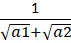Multiply numerator and denominator by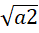-We get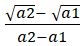. We know, a2 - a1 = d Similarly, repeat the same for the entire series. We get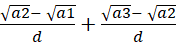+ ..... +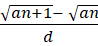=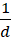$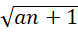-)
We do not know the value of d.
We know an+1 = a1 + nd
an+1 - a1 = nd
d =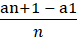=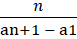So, our expression =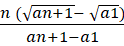=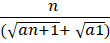The question is "If a1, a2, ......... are in A.P , $$frac{1}{\sqrt{a_1} + \sqrt{a_2}}$ + $\frac{1}{\sqrt{a_2} + \sqrt{a_3}}$ + ......... + $\frac{1}{\sqrt{a_n} + \sqrt{a_{n+1}}}$ then , is equal to" ##### Hence, the answer is $\frac{n}{\sqrt{a_1} + \sqrt{a_{n+1}}}$ Choice A is the correct answer. ###### Best CAT Online Coaching Try upto 40 hours for free Learn from the best! ###### Prepare for CAT 2023 with 2IIM's Daily Preparation Schedule ###### Know all about CAT Exam Syllabus and what to expect in CAT ###### Already have an Account? ###### CAT Coaching in ChennaiCAT 2023 Classroom Batches Starting Now! @Gopalapuram ###### Best CAT Coaching in Chennai Introductory offer of 5000/- Attend a Demo Class ###### Best Indore IPM & Rohtak IPM CoachingSignup and sample 9 full classes for free. Register now! ##### Where is 2IIM located? 2IIM Online CAT Coaching A Fermat Education Initiative, 58/16, Indira Gandhi Street, Kaveri Rangan Nagar, Saligramam, Chennai 600 093 ##### How to reach 2IIM? Mobile:$91) 99626 48484 / 94459 38484
WhatsApp: WhatsApp Now
Email: info@2iim.com